# Classes and Objects in ECMAScript

#### View more Tutorials: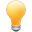Follow us on our fanpages to receive notifications every time there are new articles.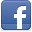Facebook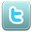Twitter

ECMAScript 5 does not have the explicit concept of Class. Instead, it simulates a class based on the two concepts such as function & prototype. Because ES5 was introduced in 2011, its syntax has been very popular so far; however, we can not deny that creating a class in such a way is hard to understand for developers. Meanwhile, the languages such as Java, C#, .. have a more modern way to create a class. The ES6 version, released in 2015, promptly patches this issue. It provides a modern syntax for defining a class.
Below, I have a Rectangle, which has 2 important properties such as width and height. We will define a class named Rectangle to simulate it with the ES6 syntax​​​​​​​​​​​​​​.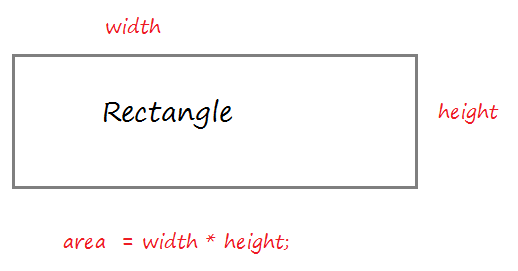Create a resource file rectangle.js:
rectangle.js
``````
// Define a class.
class Rectangle  {

// Contructor with 2 parameters.
// (Used to create instance)
// this.width refers to the width property of the class
constructor (width = 5 , height = 10)  {
this.width = width;
this.height = height;
}

// A method calculates the area of the rectangle.
getArea() {
var area = this.width * this.height
return area
}

}

// Create an object of Rectangle via Constructor.
var rect = new Rectangle(3, 5);

console.log("Height "+ rect.height);
console.log("Width "+ rect.width);

// Call method
let area = rect.getArea();
console.log("Area "+ area );
``````
Running the example:
``````
Height 5
Width 3
Area 15
``````
What will happen when you create an object from the constructor of the class?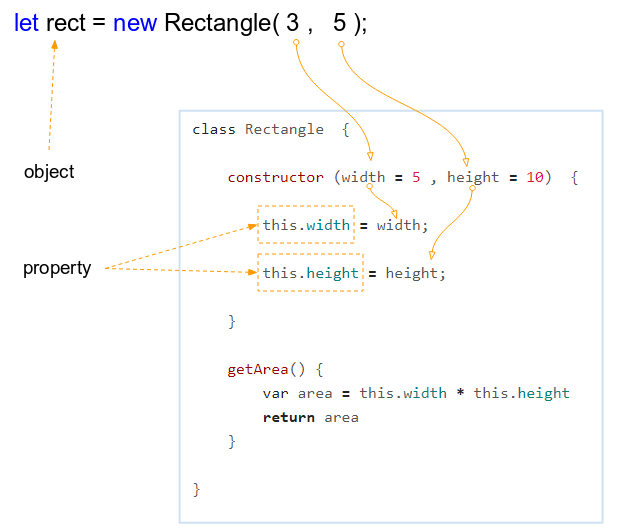When you call the Constructor of class, a new object will be created and the properties of the object will be assigned values from parameters.
In the ECMAScript, each class has at most only one constructor. Like a function, the parameters of the constructor can also have a default value. So you can create objects in different ways.
``````
// width = 3, height = 5
let rect = new Rectangle(3, 5);

// width = 15, height = 10 (Default)
let rect2 = new Rectangle(15);

// width = 5 (Default), height = 50
let rect3 = new Rectangle(undefined, 50);

// width = 5 (Default), height = 10 (Default)
let rect4 = new Rectangle();

``````

## 2- Getter & Setter

Before giving the concepts of Getter & Setter, let's analyze a situation:
Assume that we have a Person class and this class has a property which is name.
Class Person
``````
class Person  {

constructor(name) {
this.name = name;
}
}
``````
And you can access the properties of the object, or assign a new value to it without having any problems.
``````
// Create an object
let person = new Person("Tom");

console.log( person.name); // Tom

// Assign new value to property name.
person.name = "Jerry"; // !!!

console.log( person.name ); // Jerry
``````
Accessing freely to a property and changing its value from the outside of the class are really dangerous. Sometimes, you want to have a property that it is not possible to access, or assign a new value to from the outside of the class. Getter/Setter allows you to create such a property.
Okay, we write the Person class again. get keyword put in front of a method without the class parameters helps create a property with the name which is the method name. This method will be called  every time when the program accesses this property.
getter-example1.js
``````

class Person  {

constructor (name)  {
// property: __name
this.__name = name;
}

// Getter of property name
get name()  {
console.log("Call getter of property 'name'!!");
return this.__name;
}

}

// ------------ TEST -----------------

let person = new Person("Tom");

console.log( person.name); // Tom

// Assign new value to property name.
person.name = "Jerry"; // Not Working!!!!

console.log( person.name); // Tom

``````
set keyword put in front of a method with one parameter of class helps create a property with the name which is the method name. This method will be called everytime the program assigns a new value to this property.
setter-example1.js
``````

class Person  {

constructor (name)  {
// property: __name
this.__name = name;
}

// Setter of property name
set name(newName)  {
console.log("Call setter of property 'name'!!");
this.__name = newName;
}

// A method
showInfo()  {
console.log("Person: " + this.__name);
}
}

// ------------ TEST -----------------

let person = new Person("Tom");

console.log( person.name); // undefined

// Set new value to property 'name' ==> Call setter
person.name = "Jerry";

person.showInfo(); // Person: Tom
``````
Example of a property with Getter & Setter:
getter-setter-example.js
``````
class Rectangle  {

constructor (width = 5 , height = 10)  {
this.__width = width;
this.height = height;
}

// Getter of property 'width'
get width()  {
return this.__width;
}

// Setter of property 'width'
set width(newWidth)  {
if(newWidth > 0) {
this.__width = newWidth;
} else {
console.log("Invalid width " + newWidth);
}
}

}

// ------------ TEST ------------------

var rect = new Rectangle(3, 5);

console.log("Height "+ rect.height); // Height: 5
console.log("Width "+ rect.width); // Width: 3

rect.width = -100;

console.log("Height "+ rect.height); // Height: 5
console.log("Width "+ rect.width); // Width: 3

rect.width = 100;

console.log("Height "+ rect.height); // Height: 5
console.log("Width "+ rect.width); // Width: 100
``````
Output:
``````
Height 5
Width 3
Invalid width -100
Height 5
Width 3
Height 5
Width 100
``````
The properties with prefixes: 2 underscores (__) are usually conventionalized by programmers not to use it outside the class. However, that convention can be broken by someone. And therefore, using such properties is dangerous.
If you want a private property, it should be named with the prefix which is hashtag (#). However, this code can only be run with the help of Babel 7 or later.
Private property
``````
class Something {

constructor(){

this.#someProperty = "test";
}

}

const instance = new Something();

console.log(instance.#someProperty); // undefined
``````
getter-example2.js
``````
class Person  {

constructor (name)  {
// Private property: #name
this.#name = name;
}

// Getter of property name
get name()  {
console.log("Call getter of property 'name'!!");
return this.#name;
}

}
``````

## 3- Static Field

The static keyword appears in the Getter or Setter declarations to help you define a static field. You can access static fields through a class name.
static fields with fixed value (unchangeable) are called constant static fields.
static-field-example1.js
``````
class Employee {

constructor (fullName, age)  {
this.fullName = fullName;
if(age < Employee.MIN_AGE || age > Employee.MAX_AGE)  {
throw "Invalid Age " + age;
}
this.age = age;
}

// A static field: MIN_AGE
static get MIN_AGE() {
return 18;
}

// A static field: MAX_AGE
static get MAX_AGE() {
if(!Employee.__MAXA)  {
Employee.__MAXA = 60;
}
return Employee.__MAXA;
}

static set MAX_AGE(newMaxAge)  {
Employee.__MAXA = newMaxAge;
}

}

// ---- TEST ---------

console.log("Mininum Age Allowed: " + Employee.MIN_AGE);
console.log("Maximum Age Allowed: " + Employee.MAX_AGE);

// Set new Maximum Age:
Employee.MAX_AGE = 65;

console.log("Maximum Age Allowed: " + Employee.MAX_AGE);

let baby = new Employee("Some Baby", 1); // Error!!

``````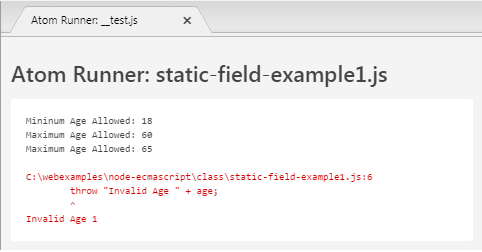You have another way for declaring a static field for a class, however, static fields created by this way will not be a Constant  because its value can change. Below is an example:
static-field-example2.js
``````
class Employee {

constructor (fullName, age)  {
this.fullName = fullName;
if(age < Employee.MIN_AGE || age > Employee.MAX_AGE)  {
throw "Invalid Age " + age;
}
this.age = age;
}
}

Employee.MIN_AGE = 18;
Employee.MAX_AGE = 60;

// ---- TEST ---------

console.log("Mininum Age Allowed: " + Employee.MIN_AGE);
console.log("Maximum Age Allowed: " + Employee.MAX_AGE);

// Set new Maximum Age:
Employee.MAX_AGE = 65;

console.log("Maximum Age Allowed: " + Employee.MAX_AGE);

let baby = new Employee("Some Baby", 1); // Error!!
``````

## 4- Static method

The Static keyword which appears in declaration of a class method helps you define a static method. You can call static methods through the class name. Static methods can not be called through the object of the class. A static method is often used as an utility function of an application.point.js
``````
class Point {

constructor(x, y) {
this.x = x;
this.y = y;
}

// Calulatesthe distance between 2 points
static distance( point1, point2) {
const dx = point1.x - point2.x;
const dy = point1.y - point2.y;

return Math.hypot(dx, dy);
}
}

// --- TEST ---
let point1 = new Point( 5, 10);
let point2 = new Point( 15, 20);

// Distance
let d = Point.distance(point1, point2);

console.log("Distance between 2 points: " + d);
``````
Output:
``````
Distance between 2 points: 14.142135623730951
``````

## 5- Object Comparison operators

In ECMAScript, when you create an object through constructor will be a real entity is created in memory, it has a specified address.

An operator assigned AA object by an BB  object does not create new entity on the memory, it's just pointing to address of AA to BB's address.=== Operator used to compare two objects that is pointing to, it returns true if the two objects refer to the same address in memory. Operators !== is used to compare the two addresses of two objects that is pointing to, it returns true if the two objects that point to two different addresses.
identify-operator-example.js
``````
// Define a class.
class Rectangle  {
constructor (width = 5 , height = 10)  {
this.width = width;
this.height = height;
}
getArea() {
var area = this.width * this.height;
return area;
}
}

// Create object: r1
let r1 = new Rectangle( 20,  10);

// Create object: r2
let r2 = new Rectangle( 20, 10);

let r3 = r1;

let  b12  =   r1 === r2; // false
let  b13  =   r1 === r3; // true

console.log("r1 === r2 ? " + b12); // false
console.log("r1 === r3 ? " + b13); // true

var bb12  = r1 !== r2; // true
var bb13  = r1 !== r3; // false

console.log("r1 !== r2 ? " + bb12); // true
console.log("r1 !== r3 ? " + bb13); // false
``````
Running the example:
``````
r1 === r2 ? false
r1 === r3 ? true
r1 !== r2 ? true
r1 !== r3 ? false
``````

## 6- Inheritance and Polymorphism

#### View more Tutorials:

Maybe you are interested

These are online courses outside the o7planning website that we introduced, which may include free or discounted courses.

•The Complete JavaScript Bootcamp
•Getting really good at JavaScript and TypeScript
•Essentials of JavaScript Practice Coding Exercises Tips
•Learn JavaScript From Scratch:Become Top Rated Web Developer
•JavaScript For Beginners - Learn JavaScript From Scratch
•Javascript for Beginners
•Learning ECMAScript 6: Moving to the New JavaScript
•JavaScript Dynamic Quiz Application from Scratch JSON AJAX
•JavaScript Intro to learning JavaScript web programming
•Byte-Sized-Chunks: Dynamic Prototypes in Javascript
•Learn ECMAScript 2015 - ES6 Best Course
•Getting started with javascript and its core concepts
•HTML CSS JavaScript: Most popular ways to code HTML CSS JS
•Quick JavaScript Core learning Course JavaScript Essentials
•*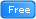* Master ECMAScript 2015 (ES6)
•Learn JavaScript Fundamentals
•** Start 3D GIS Web Development in JavaScript
•Learning JavaScript Programming Tutorial. A Definitive Guide
•2D Game Development With HTML5 Canvas, JS - Tic Tac Toe Game
•JavaScript for Beginning Web Developers
•The Web Development Course: HTML5, CSS3, JavaScript
•JavaScript in 55 Minutes
•The complete beginner JavaScript ES5, ES6 and JQuery Course
•JavaScript in Action JavaScript Projects
•** Introductory To JavaScript - Learn The Basics of JavaScript